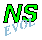## Bessel monopole encounters a circular

Details on the computations

```Initialisation
Monopole:    Bessel monopole of radius 0.5 and strength 4
centred at (+3,-3)
Tracers:     single tracer point at the centre of the vortex
Computation: domain: X = (-10, ..., 10), Y = (-10, ..., 10)
no. of grid points from wall to wall: (257, 257)
boundary conditions: stress-free along all walls
standard Arakawa scheme used
rotation effects: beta = 0.3, gamma = 0
and: f0 = 0, +3 or -3
bottom topography: circular mountain at the origin
with a half cosine profile.
max. height h_max = 0.4, radius R = 1.5
solver for streamfunction: NAG
viscosity: nu = 10^-3 (i.e. Re=1000 at T=0)
time step: dt = 0.05
final time: T = 50
```

Bessel monopole encounters a circular mountain that the origin:
<=== with f0=0: equatorial case
<=== with f0=+3: northern hemisphere
<=== with f0=-3: southern hemisphere